# Geometric Sequences and Sums

## Sequence

A Sequence is a set of things (usually numbers) that are in order.## Geometric Sequences

In a Geometric Sequence each term is found by multiplying the previous term by a constant.

### Example:

 1, 2, 4, 8, 16, 32, 64, 128, 256, ...

This sequence has a factor of 2 between each number.

Each term (except the first term) is found by multiplying the previous term by 2.In General we write a Geometric Sequence like this:

{a, ar, ar2, ar3, ... }

where:

• a is the first term, and
• r is the factor between the terms (called the "common ratio")

### Example: {1,2,4,8,...}

The sequence starts at 1 and doubles each time, so

• a=1 (the first term)
• r=2 (the "common ratio" between terms is a doubling)

And we get:

{a, ar, ar2, ar3, ... }

= {1, 1×2, 1×22, 1×23, ... }

= {1, 2, 4, 8, ... }

But be careful, r should not be 0:

• When r=0, we get the sequence {a,0,0,...} which is not geometric

## The Rule

We can also calculate any term using the Rule:

xn = ar(n-1)

(We use "n-1" because ar0 is for the 1st term)

### Example:

 10, 30, 90, 270, 810, 2430, ...

This sequence has a factor of 3 between each number.

The values of a and r are:

• a = 10 (the first term)
• r = 3 (the "common ratio")

The Rule for any term is:

xn = 10 × 3(n-1)

So, the 4th term is:

x4 = 10×3(4-1) = 10×33 = 10×27 = 270

And the 10th term is:

x10 = 10×3(10-1) = 10×39 = 10×19683 = 196830

A Geometric Sequence can also have smaller and smaller values:

### Example:

 4, 2, 1, 0.5, 0.25, ...

This sequence has a factor of 0.5 (a half) between each number.

Its Rule is xn = 4 × (0.5)n-1

## Why "Geometric" Sequence?

Because it is like increasing the dimensions in geometry: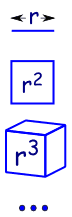a line is 1-dimensional and has a length of r in 2 dimensions a square has an area of r2 in 3 dimensions a cube has volume r3 etc (yes we can have 4 and more dimensions in mathematics).

Geometric Sequences are sometimes called Geometric Progressions (G.P.’s)

## Summing a Geometric Series

To sum these:

a + ar + ar2 + ... + ar(n-1)

(Each term is ark, where k starts at 0 and goes up to n-1)

We can use this handy formula:a is the first term
r is the "common ratio" between terms
n is the number of terms

What is that funny Σ symbol? It is called Sigma Notation(called Sigma) means "sum up"

And below and above it are shown the starting and ending values:It says "Sum up n where n goes from 1 to 4. Answer=10

The formula is easy to use ... just "plug in" the values of a, r and n

### Example: Sum the first 4 terms of

 10, 30, 90, 270, 810, 2430, ...

This sequence has a factor of 3 between each number.

The values of a, r and n are:

• a = 10 (the first term)
• r = 3 (the "common ratio")
• n = 4 (we want to sum the first 4 terms)

So:Becomes:You can check it yourself:

10 + 30 + 90 + 270 = 400

And, yes, it is easier to just add them in this example, as there are only 4 terms. But imagine adding 50 terms ... then the formula is much easier.

## Using the Formula

Let's see the formula in action:

### Example: Grains of Rice on a Chess BoardOn the page Binary Digits we give an example of grains of rice on a chess board. The question is asked:

When we place rice on a chess board:

• 1 grain on the first square,
• 2 grains on the second square,
• 4 grains on the third and so on,
• ...

... doubling the grains of rice on each square ...

... how many grains of rice in total?

So we have:

• a = 1 (the first term)
• r = 2 (doubles each time)
• n = 64 (64 squares on a chess board)

So:Becomes:= 1−264−1 = 264 − 1

= 18,446,744,073,709,551,615

Which was exactly the result we got on the Binary Digits page (thank goodness!)

And another example, this time with r less than 1:

### { 1/2, 1/4, 1/8, 1/16, ... }

The values of a, r and n are:

• a = ½ (the first term)
• r = ½ (halves each time)
• n = 10 (10 terms to add)

So:Becomes: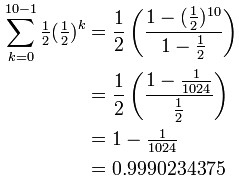Very close to 1.

(Question: if we continue to increase n, what happens?)

## Why Does the Formula Work?

Let's see why the formula works, because we get to use an interesting "trick" which is worth knowing.

First, call the whole sum "S":  S = a + ar + ar2 + ... + ar(n−2)+ ar(n−1)
Next, multiply S by r:S·r = ar + ar2 + ar3 + ... + ar(n−1) + arn

Notice that S and S·r are similar?

Now subtract them!Wow! All the terms in the middle neatly cancel out.
(Which is a neat trick)

By subtracting S·r from S we get a simple result:

S − S·r = a − arn

Let's rearrange it to find S:

Factor out S and a:S(1r) = a(1rn)
Divide by (1−r):S = a(1rn) (1r)

Which is our formula (ta-da!):## Infinite Geometric Series

So what happens when n goes to infinity?

We can use this formula: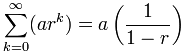But be careful:

r must be between (but not including) −1 and 1

and r should not be 0 because the sequence {a,0,0,...} is not geometric

So our infnite geometric series has a finite sum when the ratio is less than 1 (and greater than −1)

Let's bring back our previous example, and see what happens:

### { 12, 14, 18, 116, ... }

We have:

• a = ½ (the first term)
• r = ½ (halves each time)

And so:= ½×1½ = 1

Yes, adding 12 + 14 + 18 + ... etc equals exactly 1.

 Don't believe me? Just look at this square: By adding up 12 + 14 + 18 + ... we end up with the whole thing!## Recurring Decimal

On another page we asked "Does 0.999... equal 1?", well, let us see if we can calculate it:

### Example: Calculate 0.999...

We can write a recurring decimal as a sum like this: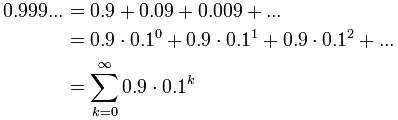And now we can use the formula: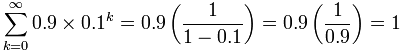Yes! 0.999... does equal 1.

So there we have it ... Geometric Sequences (and their sums) can do all sorts of amazing and powerful things.